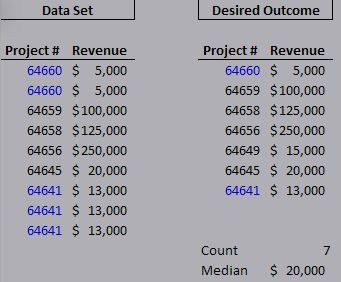cancel
Showing results for
Did you mean:Helper IV

## Duplicates - Need help with Syntax!

Hi all,

I am trying to figure out how to calculate the following count and median for a data set with recurring rows. What would the measure syntax be to achieve the results on the bottom right of the image? Thanks in advance!1 ACCEPTED SOLUTIONCommunity Champion

You can create a summarized table

`SumTable = SUMMARIZE(Table2, Table2[ProjNbr], "Revenue", MAX(Table2[Revenue])) `

For the count, you don't have to create the table explicitly:

```Cnt =
COUNTROWS (
SUMMARIZE ( Table2, Table2[ProjNbr], "Revenue", MAX ( Table2[Revenue] ) )
)orCnt = COUNTROWS (SumTable)```

But for the median I don't think you can avoid creating the table as MEDIAN requires a column name

`Mdn = MEDIAN(SumTable[Revenue])`

Hope this helps.

David

2 REPLIES 2Community Champion

You can create a summarized table

`SumTable = SUMMARIZE(Table2, Table2[ProjNbr], "Revenue", MAX(Table2[Revenue])) `

For the count, you don't have to create the table explicitly:

```Cnt =
COUNTROWS (
SUMMARIZE ( Table2, Table2[ProjNbr], "Revenue", MAX ( Table2[Revenue] ) )
)orCnt = COUNTROWS (SumTable)```

But for the median I don't think you can avoid creating the table as MEDIAN requires a column name

`Mdn = MEDIAN(SumTable[Revenue])`

Hope this helps.

DavidHelper IV

Thanks, David. That was extremely helpful!